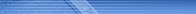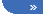Look RS232 > RS232C Hardware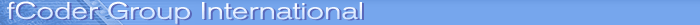fCoder SIA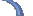Look RS232DownloadOrderSupportCookie Policy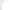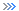Look RS232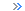DownloadOrderSupportTestimonialsFAQOnline helpModem ProgrammingSerial portSerial CommunicationSerial portSerial vs ParallelTransmition methodRS232 HardwareInterface RS232CHistory RS232CLikely problems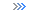Hardware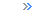Hardware PropertiesBit RatesDTE/DCE SpeedsFlow controlThe UARTTypes of UARTsNull modemsLoop Back Plug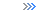Registers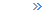Port AddressesTable of registersDLABIERIIRFCRLCRLSRMCRScratch RegisterInterfacing devicesWaveformsLevel converters8250UARTsMicrocontrollersProgrammingAbout ProgrammingOpen portSetup COM-portTimeOutsPurge COM-portWork with DCBRead and WriteEventAddingEnumportsStructureDCBCOMSTATOVERLAPPEDCOMMTIMEOUTSPORT_INFO_*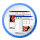COMMTIMEOUTSThe COMMTIMEOUTS structure is the second binding structure for the port setting. It detects the parameters of timeouts when receiving/transmitting data.

The members of the COMMTIMEOUTS structure have the following meaning:

DWORD ReadIntervalTimeout - sets max period of time (in milliseconds) allowed between two sequential characters being read from the line of commutation. During the 'read' operation the time period countdown takes its start when the first character is received. When the interval between two sequential characters exceeds the given value the 'read' operation finishes and all the data accumulated in the buffer are transmitted to the programme. Zero value of this member indicates that this timeout isn't used.

DWORD ReadTotalTimeoutMultiplier- sets the multiplier (in milliseconds) used to calculate general timeout of the 'read' operation. In every case this value is multiplied by the quantity of the characters requested for reading.

DWORD ReadTotalTimeoutConstant - sets a constant (in milliseconds) used to calculate the general timeout of the operation. In case of every 'read' operation this value is added to the result of multiplying ReadTotalTimeoutMultiplier by the quantity of the characters requested for the reading. ReadTotalTimeoutMultiplier and ReadTotalTimeoutConstant mean that general timeout for 'read' operation isn't used.

DWORD WriteTotalTimeoutMultiplier - sets the multiplier (in milliseconds) used to calculate general timeout of the 'write' operation. In every case this value is multiplied by the quantity of the characters being written.

DWORD WriteTotalTimeoutConstant - sets a constant (in milliseconds) used to calculate the general timeout of the 'write' operation. In case of every 'write' operation this value is added to the result of multiplying WriteTotalTimeoutMultiplier by the quantity of the characters being written. Zero value of the WriteTotalTimeoutMultiplier and WriteTotalTimeoutConstant members indicates that general timeout for the 'write' operation isn't used.

A bit more about timeouts. Imagine that we read 50 characters at a rate of 9 600 bps. If 8 bits per character, parity complement and one stop bit are used, then there'll be 11 bits (including the start bit) per character in the physical line. So 50 characters at a rate 9 600 bit/s will be received as

50x11x9600=0,0572916 sec

or approximately 57,3 milliseconds, if there's a zero interval between the reception of sequential characters. If the interval between the characters is about a half time of one character transmission, i.e. 0,5 milliseconds, the reception time is calculated as

50x11x9600+49x0,0005=0,0817916 sec

or approximately 82 milliseconds. If the reading process has amounted to 82 milliseconds, we can suppose there's been an external device error and stop the 'read' operation to evade the programme buzz. This is the general timeout of the 'read' operation. The general timeout of the 'write' operation is quite similar to it.

Below is the formula to be used when calculating, for example, the general timeout of the 'read' operation: